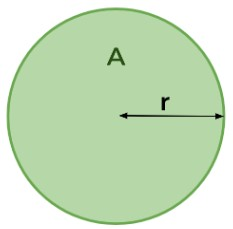# Circle Perimeter Calculator

Enter radius or diameter and click the calculate button to find the perimeter using the circle perimeter calculator

Give Us Feedback

## Circle Perimeter Calculator

Use the perimeter of a circle calculator to find the perimeter of a circle. This advanced perimeter tool gives the steps of the circle Perimeter calculation as well.

## What is the Circle Perimeter?

The perimeter of a circle, often referred to as its circumference, is the distance around it. It provides insight into the length of the boundary enclosing the circular area. Calculating the circumference helps in understanding the spatial extent of the circle in a two-dimensional plane.## Circle Perimeter Formula:

The formula used to find the perimeter of a circle is given below;

Circle perimeter = C = 2πr

Here π is the mathematical constant pi. Its value is 3.14.

Note: r is not equal to the diameter of the circle i.e. 2r.

## How to find the circle perimeter?

The allmath’s calculator to find the circle perimeter gives 100 percent accurate results. Other than that, there is a manual method.

2. Multiply the radius by 2.
3. Multiply the value with pi.
4. The final value is the perimeter of the circle.

Example:

A circle has a radius of 7cm. Find its perimeter.

Solution:

Step 1: Put in the formula.

Circle Perimeter = 2πr

Circle Perimeter = 2π(7)

Circle Perimeter = π(14)

Step 3: Solve.

Circle Perimeter = (3.14)(14)
Circle Perimeter = 43.96 cm

### Math Tools